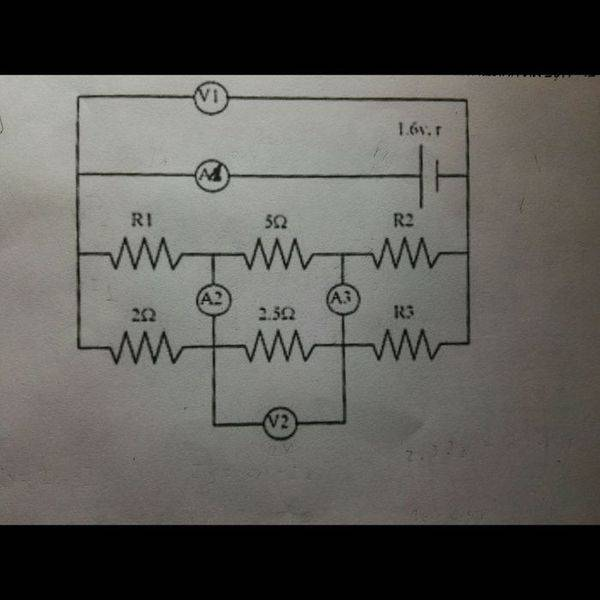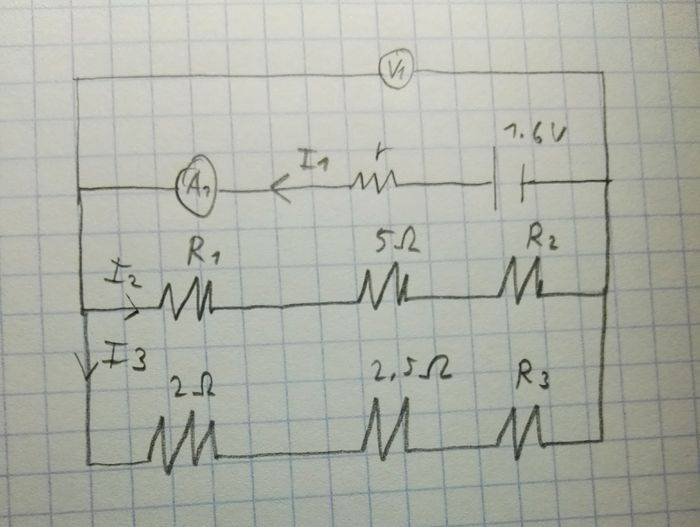# Finding Resistance

## Homework Statement

The two voltmeters have very large resistance, the 3 ampere meter have resistance of ##0.5\Omega## each one.
##V_1## show twice as much voltage as ##V_2##, ##A_1## shows ##0.4A## and ##A_2=A_3=0A##

a. Find ##R_2##
b. Find the internal resistance of the battery## Homework Equations

KVL and KCL
Thevenin and Norton
Pi-T

## The Attempt at a Solution

[/B]
I did not saw a reason to use Thevenin or Norton, because ##A_2=A_3=0A## I can look at the circuit like this:1) ##0.4=I_2+I_3##
2) ##1.6-0.4r-R_1*I_2-5I_2-R_2*I_2=0##
3) ##1.6-0.4r-2I_3-2.5I_3-R_3*I_3=0##
4) ##2V_2=V1\rightarrow 1.6-0.4r=5I_3##
5) ##A_2=0A## so there is also not voltage so ##R_1*I_2+2*I_3=0##
6)##A_3=0A## so there is also not voltage so ##R_2*I_2+R_3*I_3=0##

But I am left with unknowns

BvU
Homework Helper
Ω Did you use the 2.5 Ω and the 5 Ω ? if there is no current in A2 and A3, what does that say about the currents through those two knowns ?

I used ##2.5 \Omega## in equation 3 and ##5 \Omega## in equation 2.

If there is no current, there is no voltage, I used this in equations 5 and 6

gneill
Mentor
You can do some more sleuthing before you start writing loop equations. As you have deduced, the zero amp readings of the ammeters A2 and A3 tell you that the potentials at the corresponding junctions of the resistor branches must be the same. That is, the two resistor branches split the potential difference across the branch in the same way in both branches. That should tell you something about the relationship between corresponding resistors in each branch (think ratios and scaling).

Further, the fact that the potential across the middle resistors is half that of V1 tells you something else about the resistors in each branch. Look at what V1 is measuring.

SammyS
Staff Emeritus
Homework Helper
Gold Member
If there is no current, there is no voltage, I used this in equations 5 and 6
5) A2=0AA_2=0A so there is also not voltage so R1∗I2+2∗I3=0R_1*I_2+2*I_3=0
6)A3=0AA_3=0A so there is also not voltage so R2∗I2+R3∗I3=0R_2*I_2+R_3*I_3=0
You have sign errors in those.

If no current passes through A2 or A3, then how do the voltage drops across the 5Ω and 2.5Ω resistors compare?

You should be able to express the current through the 2.5Ω resistor (I3) in terms of V2 .

You can do some more sleuthing before you start writing loop equations. As you have deduced, the zero amp readings of the ammeters A2 and A3 tell you that the potentials at the corresponding junctions of the resistor branches must be the same. That is, the two resistor branches split the potential difference across the branch in the same way in both branches. That should tell you something about the relationship between corresponding resistors in each branch (think ratios and scaling).

Further, the fact that the potential across the middle resistors is half that of V1 tells you something else about the resistors in each branch. Look at what V1 is measuring.
So I can say that ##0.4A*\frac{R_1}{R_1+2}##?

As for the voltmeter I can say that ##2*I_3+2*2.5*I_3+R_3*I_3=1.6-0.4r##?

gneill
Mentor
So I can say that ##0.4A*\frac{R_1}{R_1+2}##?
That's not an equation...
As for the voltmeter I can say that ##2*I_3+2*2.5*I_3+R_3*I_3=1.6-0.4r##?
No equations yet! What is the voltmeter V1 across? It reads the branch voltage for ALL branches, right? They are parallel branches.

Back to the resistors:
If both branches divide the branch voltage in the same way, then all the resistor ratios in one branch must be duplicated in the other. For example, in the bottom branch the first two resistors have ratio 2/2.5. Then the middle branch's first two resistors must have the same ratio. So R1/5 = 2/2.5. What's R1? In fact, the resistors in one branch must be related to the corresponding resistors in the other branch by a single scaling factor (what is it?).

Note that V1 is the whole branch voltage, and the middle resistor of each branch is said to drop half of it. What does that tell you about the the rest of the resistance in each branch? (Hint: Remember how a voltage divider works).